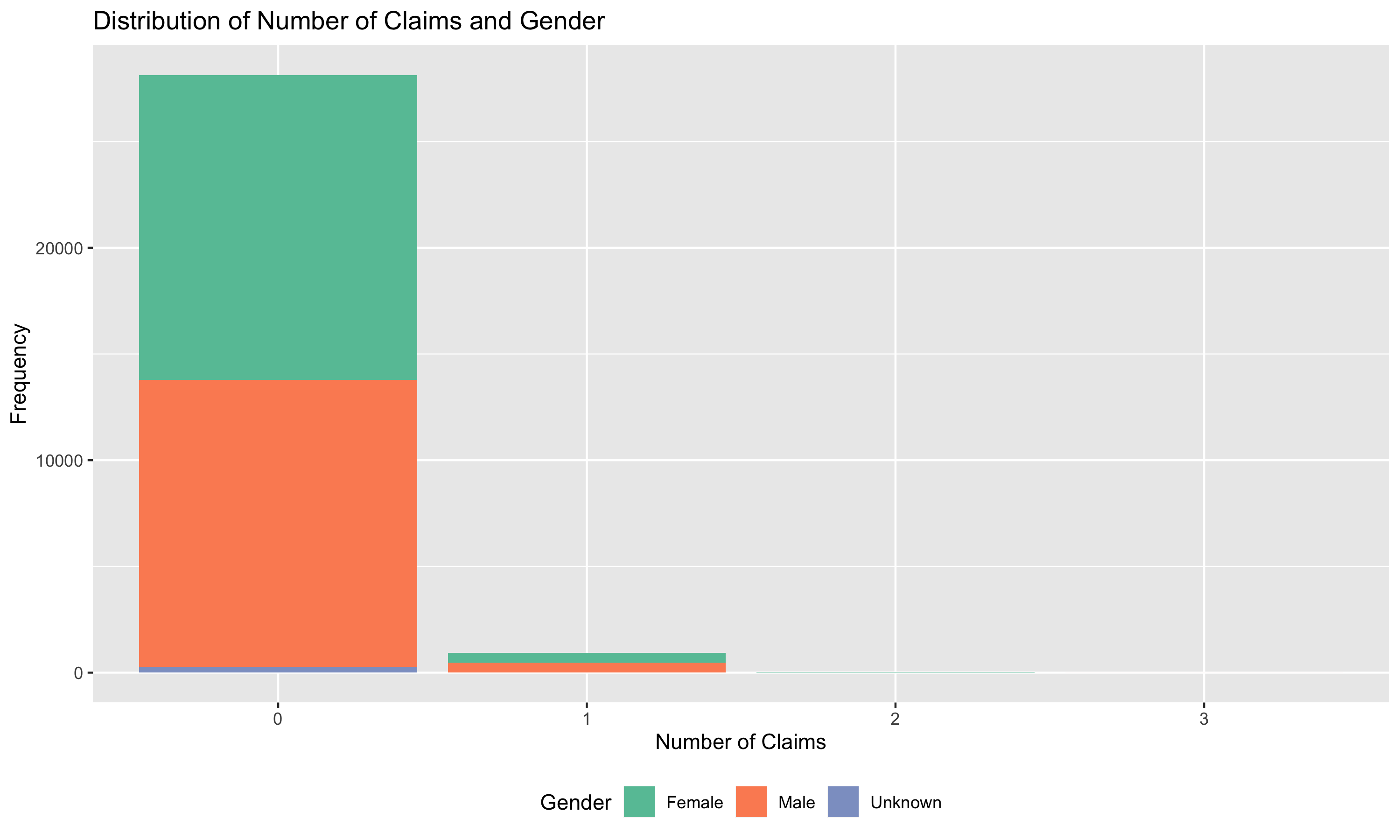Jean-Philippe Boucher, Université du Québec À Montréal (🐦 @J_P_Boucher)

Arthur Charpentier, Université du Québec À Montréal (🐦 @freakonometrics)

Ewen Gallic, Aix-Marseille Université (🐦 @3wen)

# 1 Duration Models

## 1.1 Composition of the portfolio

Analyse the composition of the portfolio: covariates, frequency by covariates.

First, load the training and testing sets, located in the following folder: `data/canada_panel/` (`CanadaPanelTrain.csv` and `CanadaPanelTest.csv`, respectively).

``library(tidyverse)``

Define a function that computes summary statistics for a vector of numerics:

• Average
• Standard deviation
• Min and Max
• Median
• Other percentiles (e.g., 10th, 25th, 75th, and 90th)

Try to account for possible `NA` values. Name the function `my_summary`.

``````#' my_summary
#' Returns a tibble with summary statistics for a numerical vector
#' @param x vector of numerics
my_summary <- function(x) {

}``````

Use the function on a single variable from the training sample:

Apply this function to multiple variables of your choice to explore the dataset and put the results in a table. You may either use a for loop, `lapply()` or `map()` (pick the option you are more comfortable with). Do not forget to add the variable name to be able to identify the variables.

Plot the distribution of claims on a barplot. You may display the distribution of gender among each claims levels (by showing the proportion of each gender within the bars). The result may look like the figure displayed below:Plot a boxplot of exposure time depending on marital status and vehicle use. Use faceting to separate the data according to vehicule use. The resulting plot should look like the one below: